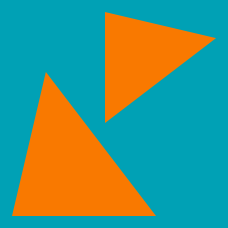Geometry

# Congruent and Similar Triangles: Level 2 Challenges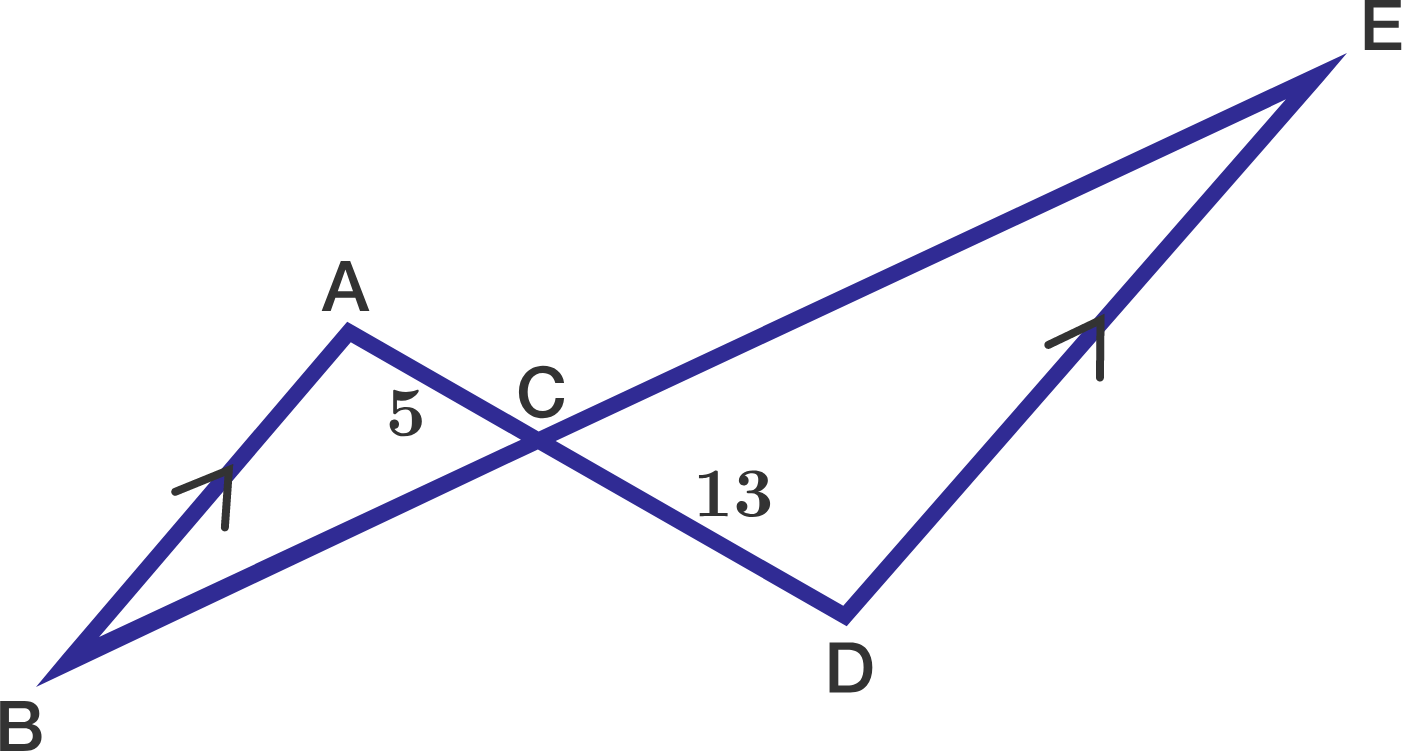$\overline{AB}$ is parallel to $\overline{DE}$, $AC=5,$ and $CD=13.$

If the area of $\triangle ABC$ is $11,$ what is the area of $\triangle CDE$ ?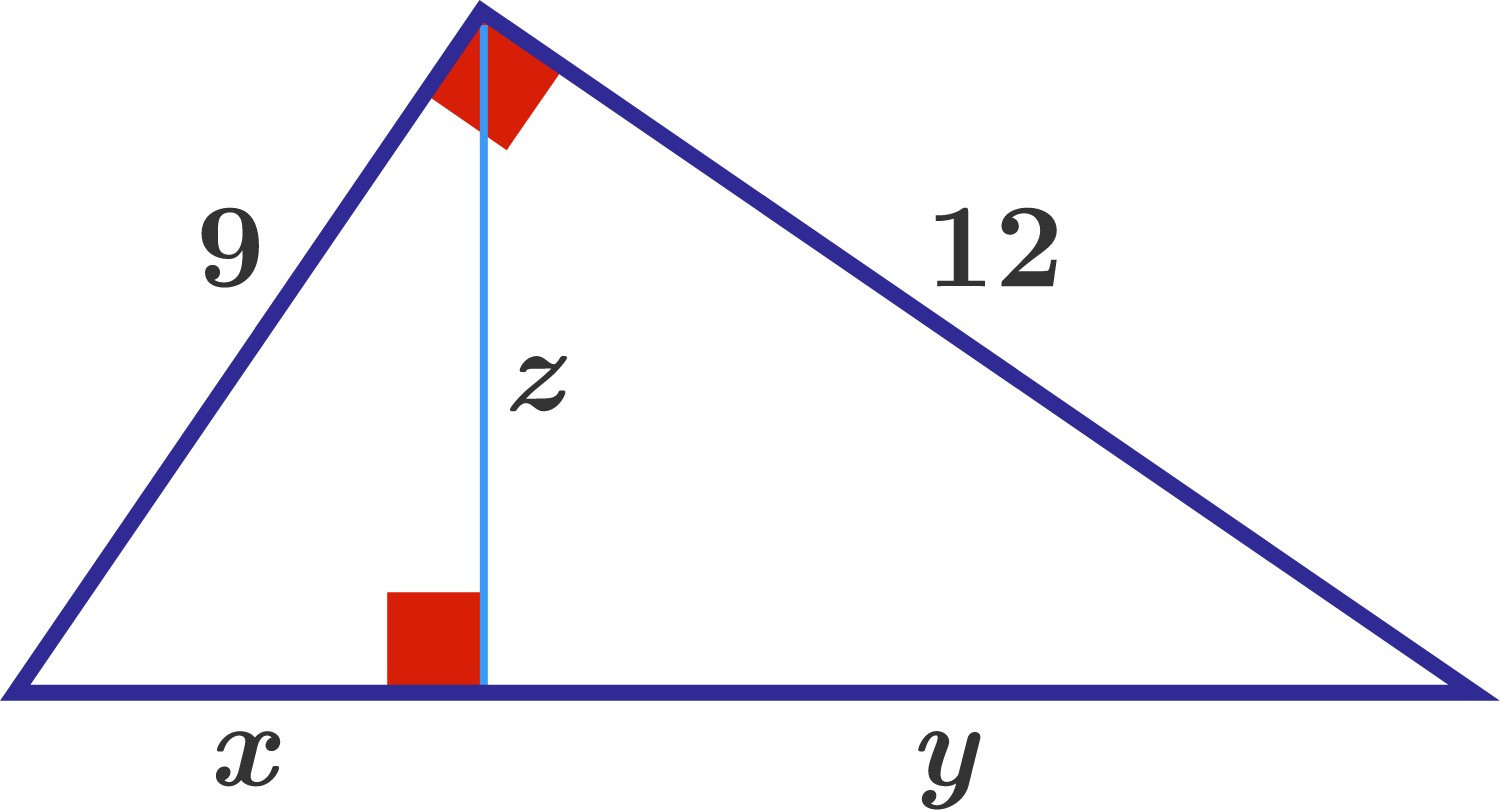In the right triangle above, a height is drawn to the hypotenuse. Find $x+y+z.$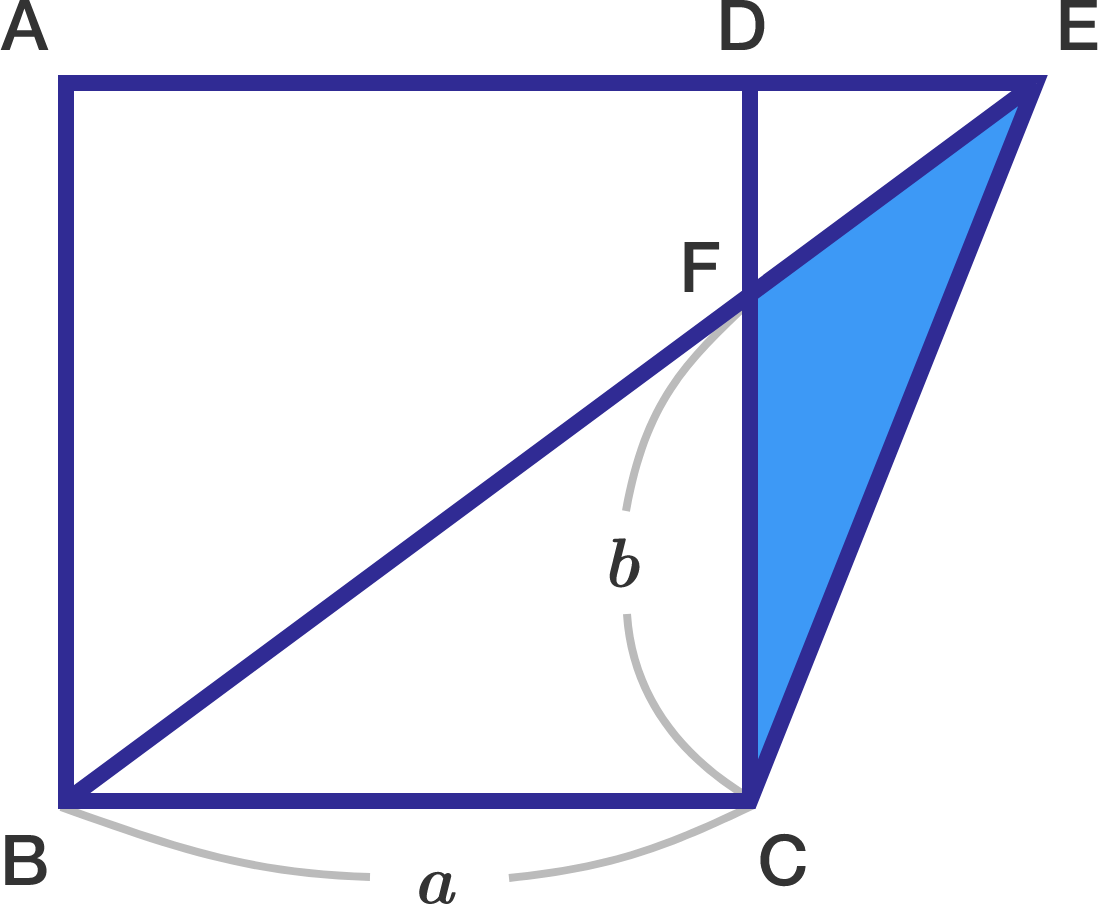In the above diagram, $\square ABCD$ is a square with side $\overline{AD}$ extended to point $E$, and $\overline{BE}$ is a straight line. If the length of $\overline{BC}$ is $\lvert \overline{BC}\rvert = a=30$ and $\lvert \overline{CF}\rvert =b= 20,$ what is the area of $\triangle CEF?$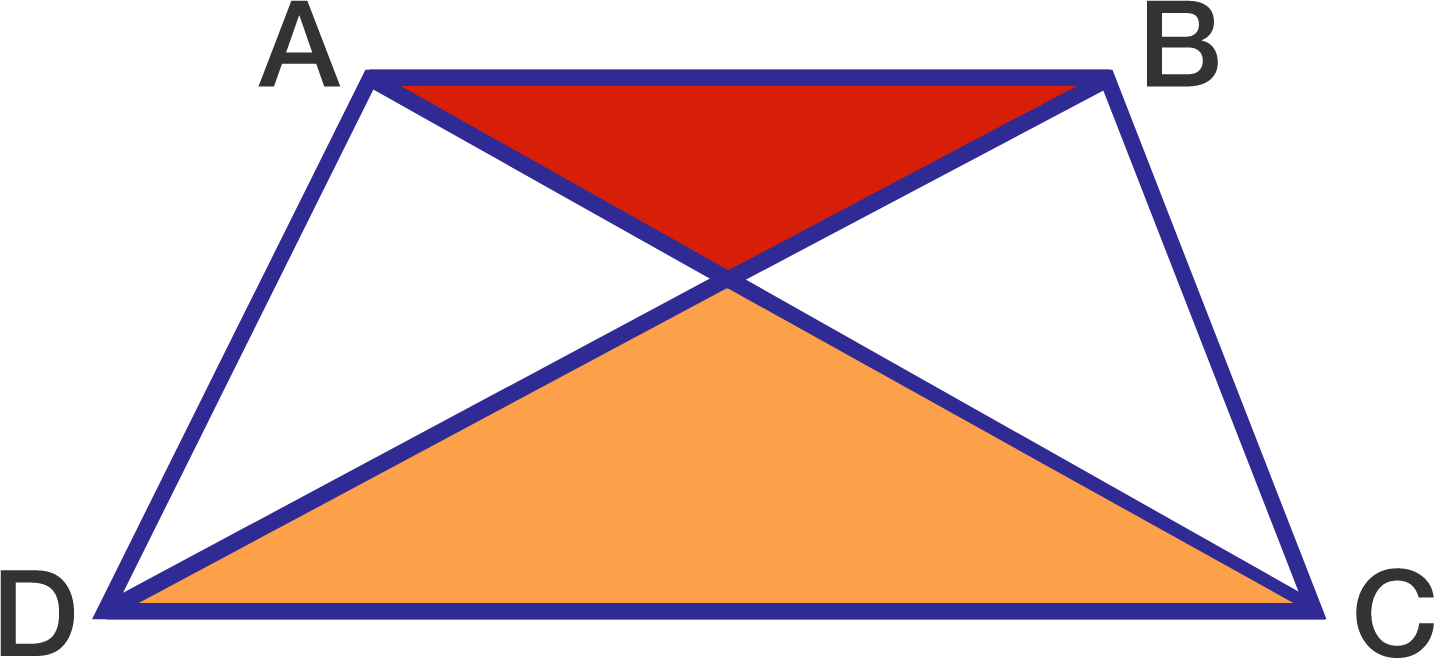The area of the red triangle is 25 and the area of the orange triangle is 49. What is the area of the trapezium $ABCD?$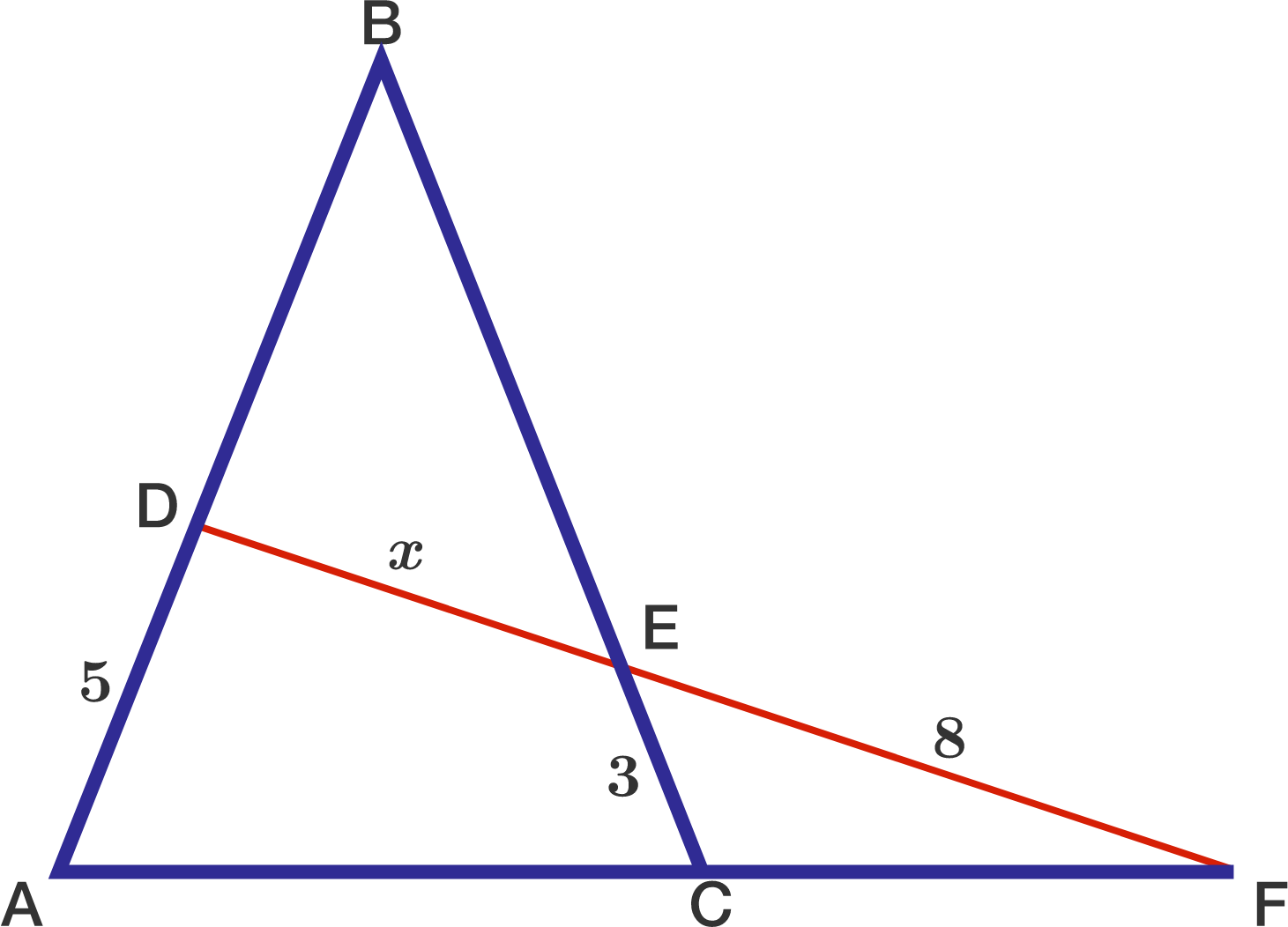The figure shows an isosceles $\triangle ABC$ with $AB = BC$. The line $DE$ cuts $AC$ extended at $F$. If $AD = 5, CE = 3,$ and $EF = 8,$ find $DE$.

×